The MAD Computer Program is a BASIC program originally published in MAD magazine No. 258 from October 1985 that renders the MAD logo, Alfred E. Neuman’s face, and Alfred E. Neuman’s motto, “What, me worry?”. This article recounts the labyrinthine journey taken to convert the MAD Computer Program into a Turing’s Maze. The result requires only about a minute of emulation on a 5½-year-old desktop PC to generate the output: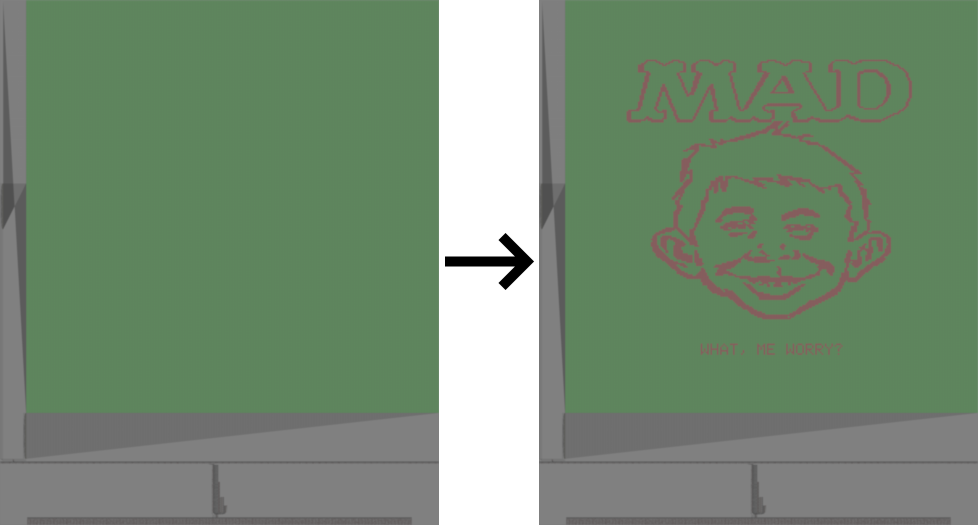Click on either image to view a full-resolution version (9,799 × 11,717 pixels). The maze file is available here.

To produce the logo and face, the MAD Computer Program draws 522 line segments. It renders thick lines by plotting pairs of horizontally adjacent pixels rather than single pixels. And once done with the logo and face, it prints the motto beneath them.

The conversion contains a 256×256 pixel display, the coordinates of the pixels comprising the motto, a line drawer circuit, and the endpoint coordinates of the line segments: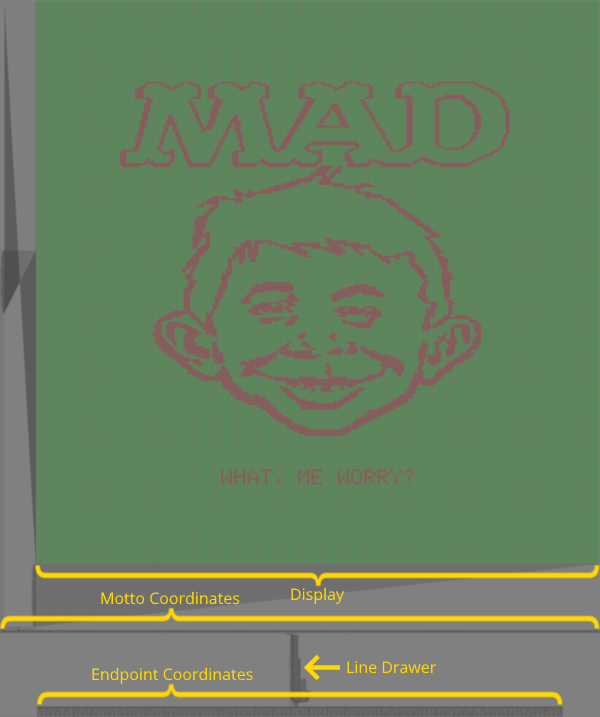As revealed in the high-level diagram below, the line drawer depends on an adder-subtractor and an equality comparator, demultiplexers drive the display, and everything shares an 8-bit bus.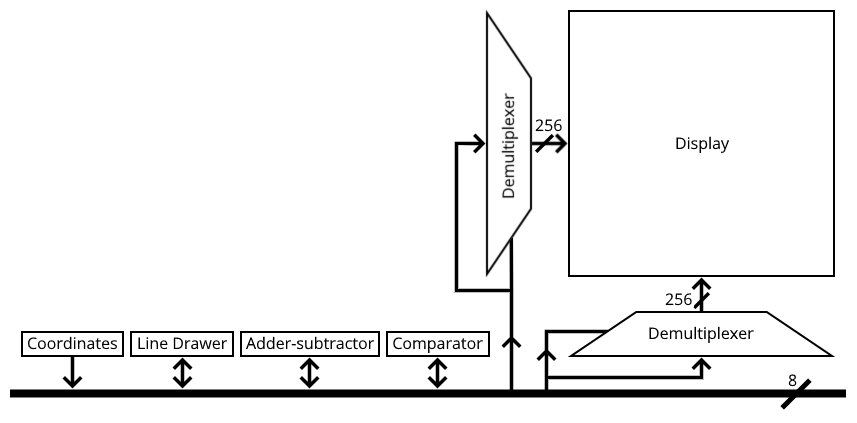The maze stores the endpoint coordinates and the motto pixel coordinates in a grid of empty and buffered-filled cells, which represent logic zeros and logic ones, respectively. The grid acts as a purely sequential microprogram. As the mouse visits the cells west-to-east and south-to-north, it transfers values into the 8-bit bus and control lines in a specific order, operating the display and the line drawer.

Here is a zoom-up of part of the endpoint coordinates grid: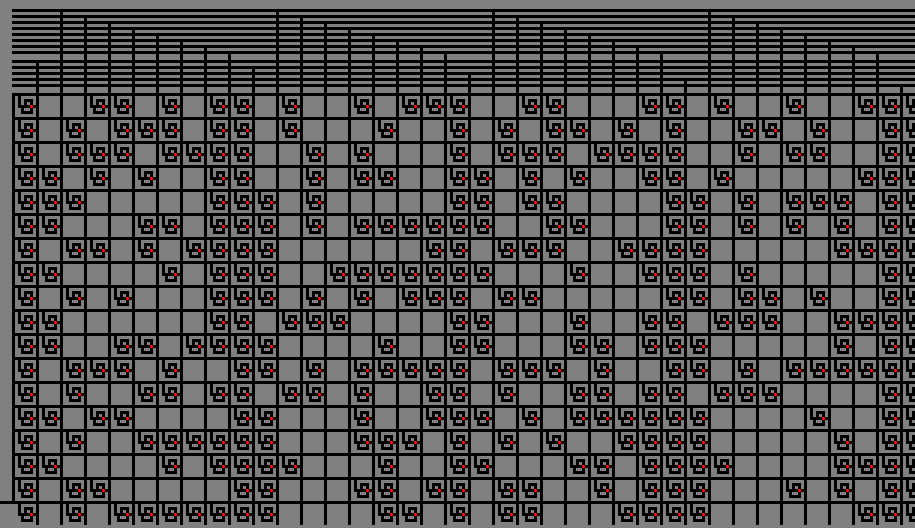The motto cells encode 5×7 pixel glyphs from the Signetics 2513 character generator chip, which is used in the Apple II, the platform graphics designer Lauretta Jones created the logo and face on, and the first of four of which the magazine article provides BASIC dialect ports, the others being the Atari 8-bit family, the Commodore 64/128, and the IBM PC.

The adder-subtractor is a truncated version of the one employed by the Mandelbrot set maze. It processes 8-bit values instead of 16-bit values: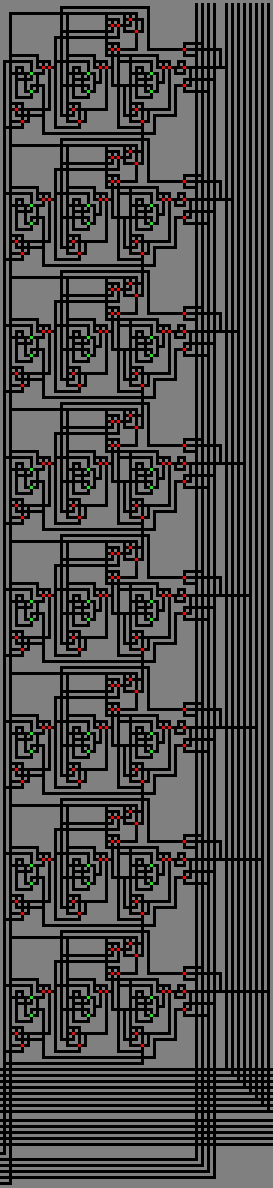The maze could determine if two 8-bit values match by subtracting them and passing their difference to an 8-input NAND gate that checks for zero. But, for performance, the maze employs a dedicated equality comparator circuit that channels input-bit pairs into XNOR gates whose outputs combine through an 8-input AND gate: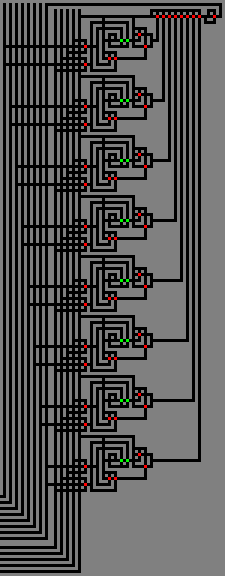The line drawer contains ten 8-bit registers:

RegisterDescription
X0First endpoint x-coordinate
Y0First endpoint y-coordinate
X1Second endpoint x-coordinate
Y1Second endpoint y-coordinate
DXLine segment run
DYLine segment rise
SXStep in x-direction
SYStep in y-direction
EInteger incremental error
E2Twice the error

The line drawer’s registers and microprogram are labeled in the image below. Note: E2 is wired such that a read performs a left-shift by 1, doubling the value on the bus.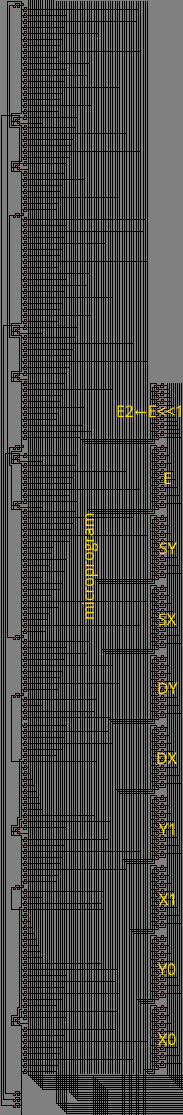The microprogram implements Bresenham's line algorithm:

```DX ← X1 − X0
if DX7 = 1 then
DX ← 0 − DX
SX ← −1
else
SX ← 1
end

DY ← Y1 − Y0
if DY7 = 1 then
SY ← −1
else
DY ← 0 − DY
SY ← 1
end

E ← DX + DY

while true do
plot(X0, Y0)
plot(X0 + 1, Y0)

if X0 = X1 then
if Y0 = Y1 then
break
end
end

E2 ← E << 1

if (E2 − DY)7 = 0 then
if X0 = X1 then
break
end
E ← E + DY
X0 ← X0 + SX
end

if (DX − E2)7 = 0 then
if Y0 = Y1 then
break
end
E ← E + DX
Y0 ← Y0 + SY
end
end
```

The line drawer plots a point by toggling a dichromatic cell of a 256×256 matrix from green to red. The image below depicts a 4×4 submatrix of the display.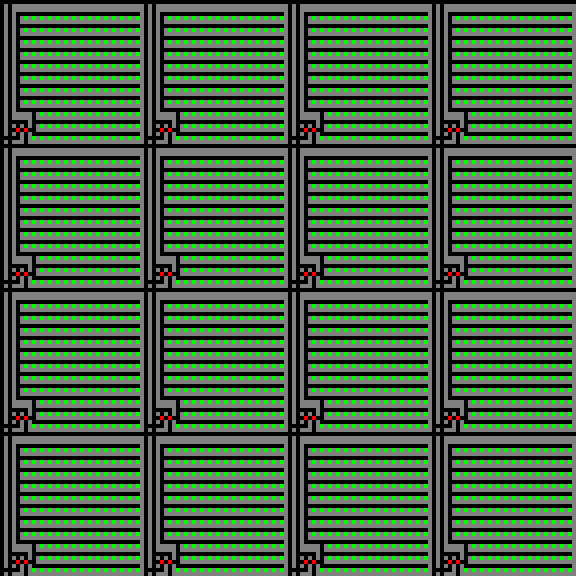The display resembles the one in the Mandelbrot set maze, except the color scheme is inverted and the cells are twice the size.